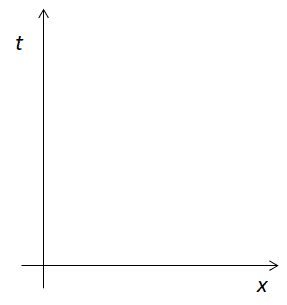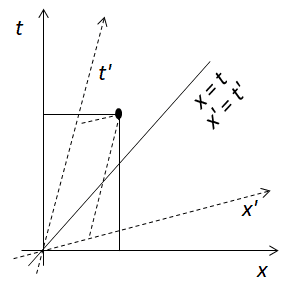# Extra dimensions

• B
andrazg
Why do physicist presenting extra dimensions with 2d creature experiencing 3d space with object falling through 2d dimension. And that, that 2d creature can see only a slice of the 3d dimension.

If time == space that means that 2d creature also experiences time because objects are moving through their plane, so the slices of the object are objects diameter through time.
So if time can be experienced in 2d dimension that means that dimensions are interconnected and not just an upgrade of one another.

The question is...What is time in 1d space?

Gold Member
2022 Award
The question is...What is time in 1d space?
Exactly the same thing it is in 2D and 3D. You can move backwards/forwards in a 1D universe and it takes time to do it.

andrazg
Exactly the same thing it is in 2D and 3D. You can move backwards/forwards in a 1D universe and it takes time to do it.

Exactly but if time is the essence of 4d that can be thought that dimensions without one another cannot exist. Because 3d without 2d and 1st is not a dimension on its own. Dimensions are essential building blocks of reality. What do you think?

Andreas

Homework Helper
Exactly but if time is the essence of 4d that can be thought that dimensions without one another cannot exist. Because 3d without 2d and 1st is not a dimension on its own. Dimensions are essential building blocks of reality. What do you think?
You are overthinking this severely.

A "dimension" in physics is something rather more mundane than is portrayed in the popular tropes. Informally, it is how many numbers you need to locate a position using a coordinate system on the space in question. A two dimensional space needs two numbers to find a place. A three dimensional map needs three. Three dimensional space plus time needs four numbers. Each "dimension" amounts to one coordinate in the coordinate system.

An individual dimension is not anything physical at all. At best, it is a feature of a particular coordinate system we use to map the space or space-time.

To get painfully technical, the dimensionality of a vector space is the minimum number of basis vectors required to span that space. https://en.wikipedia.org/wiki/Vector_space#Basis_and_dimension

•Klystron and sophiecentaur
Gold Member
Exactly but if time is the essence of 4d
Time is not "the fourth dimension" of popular literature so you can't discuss it validly in this way. @jbriggs444 put it in a more technical way but the conclusion is the same.
There's the risk that you can spend a lot of time reading opinions about what time is and emerge none the wiser.•jbriggs444
Gold Member
2022 Award
You are overthinking this severely.#### Attachments

•Khatti and Klystron
Mentor
If time == space
Time does not equal space. The dimensions of spacetime is more correctly described by the metric signature. For this universe it is (-+++) meaning one dimension of time and three dimensions of space. For “flatland” it would be (-++) meaning one dimension of time and two dimensions of space. A signature of (++) would be a two dimensions of space with no time. And so forth

•sophiecentaur
Gold Member
Time does not equal space. The dimensions of spacetime is more correctly described by the metric signature. For this universe it is (-+++) meaning one dimension of time and three dimensions of space. For “flatland” it would be (-++) meaning one dimension of time and two dimensions of space. A signature of (++) would be a two dimensions of space with no time. And so forth
I suppose that the existence of that form of description does not imply that any other arrangements than (-+++) have any reality. You could just as easily suggest a possible (--+) , which would knock 'Flatland' into a cocked hat.

Homework Helper
I suppose that the existence of that form of description does not imply that any other arrangements than (-+++) have any reality. You could just as easily suggest a possible (--+) , which would knock 'Flatland' into a cocked hat.
--+ is isomorphic with -++. It's just a re-labeling and a sign convention inversion.

Gold Member
--+ is isomorphic with -++. It's just a re-labeling and a sign convention inversion.
OK then - how about (--++) ?

Gold Member
Andreas, This wikipedia entry found by searching for "fourth dimensional space" may help answer your questions concerning space-time and additional dimensions. The "2D creature" analogies you mention, popularized in Edwin Abbott's math novel Flatland, refer to Euclidean space, emphasized in the attached article.

Space-time involves non-Euclidean Reimann vector space. While some of the the algebra looks similar, the geometry is quite different. https://en.wikipedia.org/wiki/Four-dimensional_space

Gold Member
getting back to the original question
What is time in 1d space?
Time is the 'second' dimension in 1-D space as shown here in a 'space-time' diagram, often used in special relativity.It gets more interesting if you consider what happens when one coordinate system (or observer) moves with respect to another. 'Classically' the one coordinate system is just flying up or down the diagram. But that would mean the speed of light changes and we know that is not true. Einstein solved the problem over 100 years ago and here's the new diagram (with speed of light =1)The primed coordinate system x',t' is the moving one. The axes scissor together according to the Lorentz transformation and an event in space time has coordinates which are smaller for t' and x' in the primed system. Light's path shown by x=t or x'=t' is the same in both coordinate systems and it has the same speed!